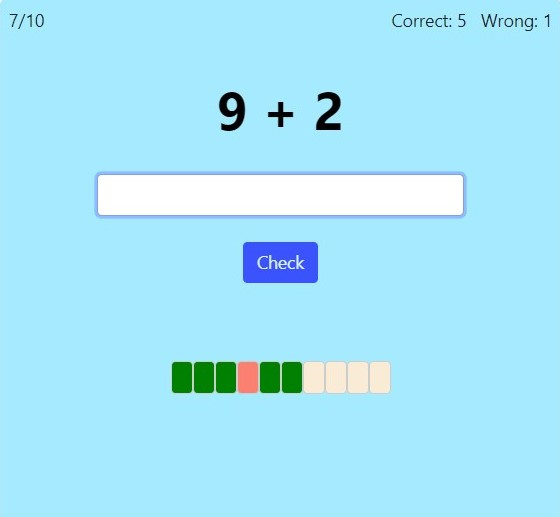On this page, you can practice the basic addition and subtraction facts with single-digit addends (such as 4 + 5, 9 + 7, 9 − 4, 12 − 8).

For first grade, choose basic facts where the sum is 10 or less, and for second grade, choose basic facts where the sum is 20 or less. Additionally you can select timed or untimed practice and the number of practice problems.

Further options for addition only include: adding with zero, doubles, doubles plus one, nine trick (or adding with 9), and the eight-trick (or adding with 8).

Screenshot:Choose the types of addition problems: